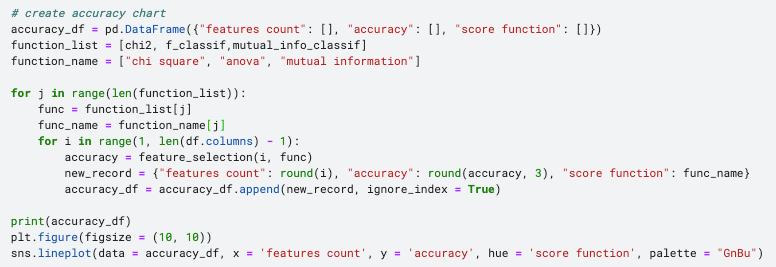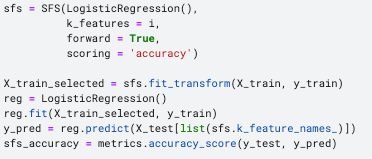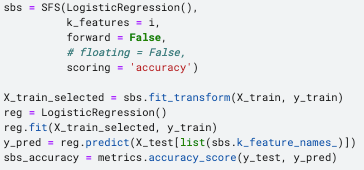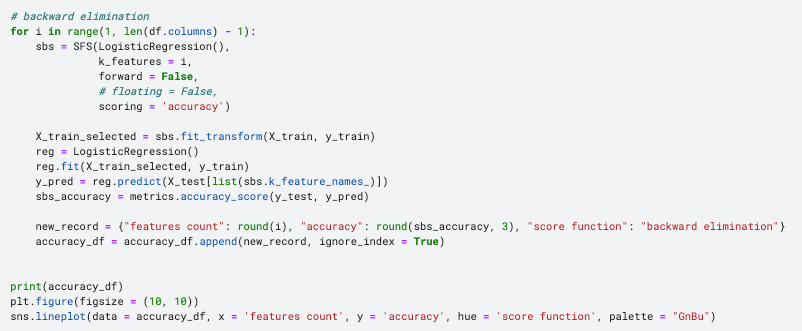top of pageSearch

# Feature Selection and EDA in Machine Learning

Updated: May 26, 2021

How to Use Data Visualization to Guide Feature SelectionIn the machine learning lifecycle, feature selection is a critical process that selects a subset of input features that would be relevant to the prediction. Including irrelevant variables, especially those with bad data quality, can often contaminate the model output.

1) avoid the curse of dimensionality, as some algorithms perform badly when high in dimensionality, e.g. general linear models, decision tree

2) reduce computational cost and the complexity that comes along with a large amount of data

3) reduce overfitting and the model is more likely to be generalized to new data

4) increase the explainability of models

In this article, we will discuss two main feature selection techniques: filter methods and wrapper methods, as well as how to use data visualization to guide decision making.

## Data Preprocessing

Before jumping into the feature selection, we should always perform data preprocessing and data transformation:

1. Load dataset and import libraries

I am using Credit Card Customer Dataset from Kaggle to predict who is more likely to get churned.

```import pandas as pd
import numpy as np
import seaborn as sns
import matplotlib.pyplot as plt
from pandas.api.types import is_string_dtype, is_numeric_dtype

Now let's have a glimpse of the raw data.2. Define the prediction: this exercise would be a classification problem, from which we predict the binary variable "Attrition_Flag" which can either be existing customers or attrited customers.

3. Examine missing data:Luckily, this dataset does not contain any missing values, but it is not always the case. If you would like to know more about how to address missing data, you may find these articles helpful.

### How to Address Common Data Quality Issues Without Code

4. Variable transformation: This process consists of encoding categorical variables and transforming all variables into the same scale. I chose label encoder and min-max scaling respectively.## Exploratory Data Analysis (EDA)

Data visualization and EDA can be great complementary tools to feature selection process, and they can be applied in the following ways:

1. Univariate Analysis: Histogram and Bar Chart help to visualize the distribution and variance of each variable

2. Correlation Analysis: Heatmap facilitates the identification of highly correlated explanatory variables and reduce collinearity

3. Bivariate Analysis: Box plot and Grouped bar chart help to spot the dependency and relationship between explanatory variables and response variable

(to have a better understanding of the data, exploratory data analysis can be performed before data transformation)

Univariate Analysis - Histogram and Bar Chart

To obtain an overview of distribution, firstly let's classify features in to categorical and numerical variables, then visualize categorical features using bar chart and numerical features using histogram. Visualizing the distribution gives suggestions on whether the data points are more dense or more spread out, hence whether the variance is low or high. Low variance features tend to contribute less to the prediction of outcome variable.Correlation Analysis - Heatmap

Some algorithms demands the absence of collinearity in explanatory variables, including logistic regression which will be used for this exercise. Therefore, eliminating highly correlated features is an essential step to avoid this potential pitfall. Correlation analysis with heatmap visualization highlights pairs of features with high correlation coefficient.As shown, it is not hard to find following pairs of highly correlated features:

• Customer_Age & Month_on_Book (0.79)

• Total_Trans_Amt & Total_Trans_Ct (0.82)

Based on this result, I dropped following variables:Bivariate Analysis - Box Plot and Grouped Bar Chart

Bivariate EDA investigate the relationship between each explanatory variable and the target variable. Categorical features and numerical variables are addressed using grouped bar chart and box plot respectively, and this exploration can further facilitate the statistical tests used in the filter methods, e.g. chi-squared and ANOVA test.

Grouped bar chart is used as the visual representation of Chi-Square AnalysisIndependent variables are set as the primary category. The target variable is set be be the secondary category using hue = "Attrition_Flag". As the result, it depicts whether the "Attrition_Flag" would vary in distribution across different level of the primary category. If two variables are independent, then we would expect the distribution to be the same across all levels.

This is the same logic as Chi-square test which calculates the difference between the observed value and expected value based on the assumption of independency. If there are no or little dependencies existing, we expect the ratio of each group of bars to be proportional to the ratio of attrited customers vs. existing customers. If the ratio is significantly different, then it suggests a high disparity between the observed value and expected value, which means high chi-square value, hence rejects the hypothesis that two variables are independent.

After plotting all category variables against the target label, I found that "Card_Category" seems to display a variation in ratio across Blue, Gold, Silver and Platinum. In the following section, we will find out if this is true according to the quantitative analysis.Box plot is used as the visual representation of ANOVA analysisBox plot displays the distributions of groups of numerical data through their quantiles. Each box shows how spread out the data is within group and putting boxes side by side indicates the difference among groups. It is aligned with ANOVA test which also analyze the degree of variance between-group compared to within-group. If the relative variability is large, for instance "Total_Revolving_Bal" and "Total_Cnt_Chng_Q4_Q1" shown below, then it may be an indication of that these features could contribute to predicting the labels. Let's find out whether this can be quantified by the ANOVA test in filter methods.fIf you would like to have a more thorough understanding of EDA, feel free to read my article on "Semi

## Feature Selection

This article introduces two types of feature selection methods: filter method and wrapper method.

The fundamental difference is that filter method evaluate the feature importance based on statistical tests such as Chi Square, ANOVA etc, whereas wrapper method iteratively assessed the performance of subsets of features based the performance of models generated by these features.

### Filter MethodsFilter methods give a score to each feature by evaluating its relationship with the dependent variable. For classification problems with categorical response variables, I am using these three major scoring functions: Chi-Square (score_func = chi2), ANOVA (score_func = f_classif), and Mutual Information (score_func = mutual_info_classif). To create a feature selection model, we need the SelectKBest() function, then specific which scoring functions to utilize and the how many variables to select.

`selection_model = SelectKBest(score_func=score_function, k=variable_counts)`

I would like to know how these two parameters, scoring function and the number of variables, would affect the accuracy of the model trained on the selected features.

Firstly, to create the carry out the feature selection and examine the performance of the model built upon it, I define a feature_selection function with following steps:

• import required libraries

• create a feature selection model based on two parameters: score_function (e.g. chi square) and variable counts (e.g. ranging from 1 to all features)

• train a logistic regression model based on selected features only

• calculates the accuracy scoreSecondly, to test how score functions and variable counts would affect the model performance, I iteratively passing different combinations of two parameters, "variable_counts" and "score_function", using the following code.Filter Methods with Data Visualization

The result was generated in a data frame format and then use line chart to demonstrate how the accuracy progress as the number of selected features grows. As shown, except mutual information method, the accuracy score stabilizes around 0.88 after reaching 8 features.Afterwards, let's investigate what is the score of each feature based on various approaches. This time we will use bar charts to visualize the scores has been allocated to features according to chi-square, anova or mutual information.As you can see, different approach scores the same feature differently, but some features always appear higher up on the list. For example, "Total_Revolving_Bal" is always among the top 3, which is aligned with the findings from the box plot in the bivariate EDA. And "Card_Category" does have high feature importance compared to other categorical variables, which can be explained by the grouped bar chart.

### Wrapper MethodsWrapper Methods find the optimal subsets of features by evaluating the performance of machine learning models trained upon these features. Since it incorporates model into the feature selection process, it requires more computational power. This article covers two main wrapper methods, forward selection and backward elimination. To perform forward selection and backward elimination, we need SequentialFeatureSelector() function which primarily requires four parameters:

1) model: for classification problem, we can use Logistic Regression, KNN etc and for regression problem, we can use linear regression etc 2) k_features: the number of features to be selected 3) forward: determine whether it is forward selection or backward elimination 4) scoring: classification problem - accuracy, precision, recall etc; regression problem - p-value, R-squared etc

Forward Selection

Forward Selection starts with no features in the model and incrementally adds one feature to the feature subset at a time. During each iteration, the new feature is chosen based on the evaluation of the model trained by the feature subset. Since the machine learning model is wrapped within the feature selection algorithm, we need to specify a model as one of the input parameters. I choose Logistic Regression for this classification problem and accuracy as the evaluation metrics. There is a slight difference in calculating the accuracy in the wrapper method compared to the filter method. Since we only fit the training set to the wrapper model, the accuracy score returned by the wrapper method itself is purely based on the training dataset. Therefore, it is necessary to train an additional model on the selected features and further evaluated based on the test set. To achieve this, I used the code below to import the required libraries, as well as create and evaluate the logistic regression model built upon the wrapper method.Backward Elimination

Simply put, it is just the opposite of the forward selection, starting with including all features to train the model. Then, features are iteratively removed from the feature subset based on whether they contribute to the model performance. Similarly, logistic regression and accuracy are used as the model and evaluation metrics correspondingly.Wrapper Methods and Data Visualization

Similar to the filter method, I enveloped both forward selection and backward elimination into a for loop, in order to examine whether the variable counts would matter to the accuracy score.As shown in the line chart, the accuracy grows rapidly when the feature counts is less than 4 and then remains stable around 0.88 afterwards.In this dataset, since there are only around 20 features in total, it may be hard for feature selection to yield any significant impact on model performance. However, it is undeniable that data visualization can help us to decide which features and how many features are suitable for the dataset or the objectives. This principle can definitely be extended to other dataset with more variables.